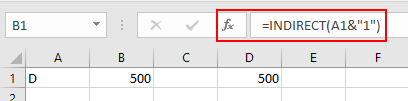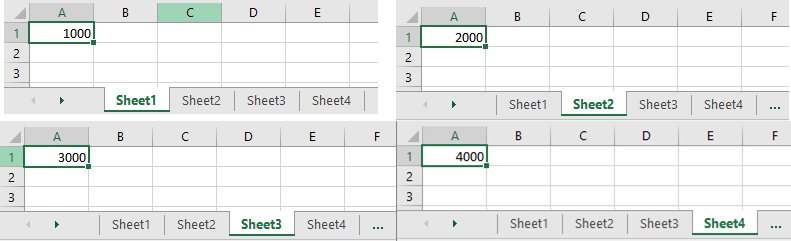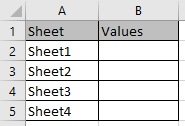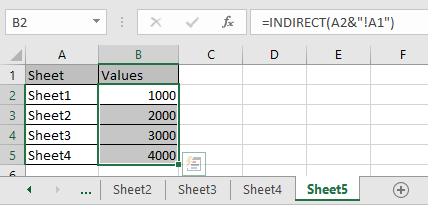# How to use the Dynamic Worksheet Reference in Excel

Dynamic worksheet reference suggests that using a value in one sheet from another.

The INDIRECT function is a cell reference function. It takes the value in the cell as address and returns the value in the address cell.
Syntax:

=INDIRECT (ref_text, [a1])

Let’s understand this function using it an example.
This is a dynamic cell reference exampleHere As you can see B1 has the Formula indicating A1 cell and Text in A1 is “D”. So the function completes the full address to find the value and returns the value from the D1 cell which is 500 into B1 cell.

Now we will learn Dynamic worksheet reference

Here we have different values in A1 cell of Sheet1, Sheet2, Sheet3 & Sheet4.Now I will get all these values in another sheet using INDIRECT function.Use the formula:

=INDIRECT(A2&"!A1")

Explanation:
Here & operator is used for joining the text to get a full address of the cell. A2 returns Sheet1 and get's join with the string “!A1” to get the full address of the worksheet with a cell reference.As you can see all the values of different sheets in another sheet.

Hope you understood how to perform dynamic worksheet reference using Indirect function and referring cell in Excel. Explore more articles on Excel function here. Please feel free to state your query or feedback for the above article.

Related Articles:

How to Get Sheet name only in Excel

How to VLOOKUP from Different Excel Sheet in Excel

Dynamic Pivot Table in Excel

How to VLOOKUP with Dynamic Col Index in Excel

How to COUNTIFS with Dynamic Criteria Range in Excel

How to perform Dynamic Named Ranges in Excel

Popular Articles:

50 Excel Shortcuts to Increase Your Productivity

How to use the VLOOKUP Function in Excel

How to use the COUNTIF function in Excel

How to use the SUMIF Function in Excel

Terms and Conditions of use

The applications/code on this site are distributed as is and without warranties or liability. In no event shall the owner of the copyrights, or the authors of the applications/code be liable for any loss of profit, any problems or any damage resulting from the use or evaluation of the applications/code.